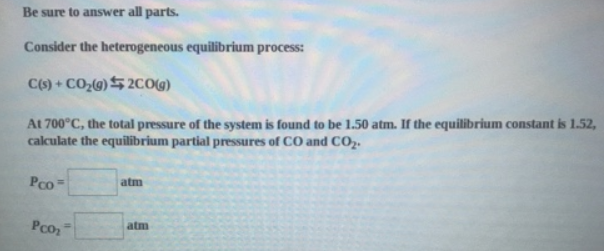Chemistry Practice Problems Equilibrium Expressions Practice Problems Solution: Be sure to answer all parts. Consider the heteroge...

# Solution: Be sure to answer all parts. Consider the heterogeneous equilibrium process: C(s) + CO2(g) ⇋ 2CO(g) At 700°C, the total pressure of the system is found to be 1.50 atm. If the equilibrium constant is 1.52, calculate the equilibrium partial pressures of CO and CO2.

###### Problem

Be sure to answer all parts.

Consider the heterogeneous equilibrium process:

C(s) + CO2(g) ⇋ 2CO(g)

At 700°C, the total pressure of the system is found to be 1.50 atm. If the equilibrium constant is 1.52, calculate the equilibrium partial pressures of CO and CO2.View Complete Written Solution

Equilibrium Expressions

Equilibrium Expressions

#### Q. An equilibrium was established for the reaction CO(g) + H2O(g) ⇌ CO2(g) + H2(g) at 500 °C. (This is an industrially important reaction for the prepara...

Solved • Tue Oct 23 2018 14:10:19 GMT-0400 (EDT)

Equilibrium Expressions

#### Q. At 409 K, this reaction has a Kc value of 0.0845. 2X(g) + 3Y(g) ⇌ 2Z(g)Calculate Kp at 409 K.[Note that the pressure is in units of atmosphere (atm).]

Solved • Tue Oct 23 2018 13:37:54 GMT-0400 (EDT)

Equilibrium Expressions

#### Q. For A + 2B ⇌ 2C           Kc = 2.39For 2C ⇌ D            Kc = 0.160Calculate the value of the equilibrium constant for the reactionD ⇌ A + 2B

Solved • Mon Oct 08 2018 14:56:15 GMT-0400 (EDT)

Equilibrium Expressions

#### Q. Write the equilibrium-constant expression for the reaction A (s) + 3B (l) ⇌ 2C (aq) + D (aq)in terms of [A], [B], [C), and [D] as needed.

Solved • Fri Oct 05 2018 14:05:20 GMT-0400 (EDT)